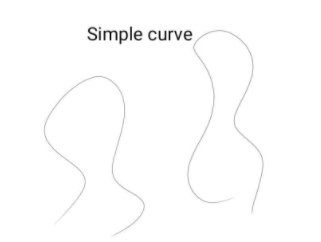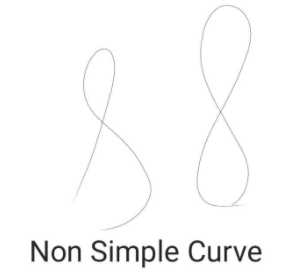Draw 2 simple and 2 non-simple curves.Verified
146.7k+ views
Hint: normal drawing of curves.

Complete step by step solution:Non simple curve is a curve that crosses itself.Additional information: A curve is a continuous and smooth flowing line without any sharp turns. One way to recognize a curve is that it bends and changes its direction at least once.
Simple Curve: A simple curve changes direction but does not cross itself while changing direction. A simple curve can be open and closed both.
Non-simple curves: A curve that crosses its own path is called a non-simple curve
A curve is made up of many small line segments joined from end to end. If you look at a curve very closely, you will see the lines.

Note: Simple Curve: A simple curve changes direction but does not cross itself while changing direction. A simple curve can be open and closed both.
Non-simple curves: A curve that crosses its own path is called a non-simple curve.
If you draw simple lines and don’t join or cross it with the body of the curve then it will also be deemed as a simple curve.
A line with curves that crosses its own body either at the end or in between its body will be deemed as a non simple curve.
There are other types of curves too. Like closed curves, non closed curves,downward curves and upward curves. These can further be also included in simple and non simple curves.